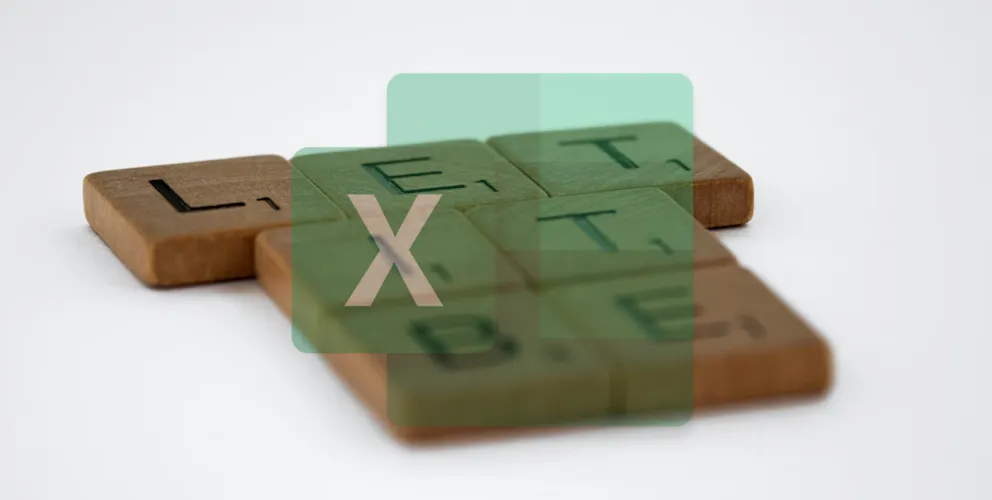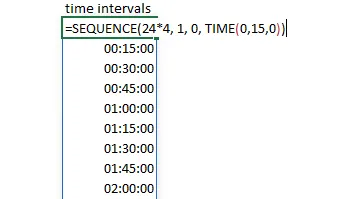# Define and use variables in Excel formula by using LET function

You can use variables in the Excel formula, and you can do that by using the function LET. Alternatively, you can use Excel capabilities to define names, but the function LET allows you to store variables inside the formula. It might be easier to maintain that in the long run.

Here is a calculation to create a list of 15-minute time intervals from this post.

`=SEQUENCE(24*4, 1, 0, TIME(0,15,0))`Imagine that I want to get a 15-minute interval list only from 8 in the morning to 8 in the evening. Luckily it is possible to use the function FILTER and get desired results, but by reusing the same thing multiple times, it looks long and cluttered. Even though I’m sometimes moving to the next line when writing Excel functions.

```=FILTER(SEQUENCE(24*4, 1, 0, TIME(0,15,0)),
(HOUR(SEQUENCE(24*4, 1, 0, TIME(0,15,0))) >= 8) *
(HOUR(SEQUENCE(24*4, 1, 0, TIME(0,15,0))) < 20))```

By using LET, I can assign a name to the calculation that is repeated multiple times like this.

```=LET(
MinIntervals,
SEQUENCE(24*4, 1, 0, TIME(0,15,0)),

FILTER(MinIntervals,
(HOUR(MinIntervals) >= 8) * (HOUR(MinIntervals) < 20)))```

By using LET, you can create multiple variables and use them further. In this case, it is not critical to do that, but for the sake of example, here is how to create an additional variable that is used in the next variable and might improve readability.

```=LET(
Step,
TIME(0,15,0),

MinIntervals,
SEQUENCE(24*4, 1, 0, Step),

FILTER(MinIntervals,
(HOUR(MinIntervals) >= 8) * (HOUR(MinIntervals) < 20)))```

Alternatively, it is possible to define names in the Formula tab. It might be better if it is necessary to reuse the same thing in multiple calculations.

Thank you for reading this post, and I invite you to take a look at other Excel-related posts in this blog.